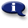# .

## Syllabus Information

Spring 2022
Jun 05,2023Use this page to maintain syllabus information, learning objectives, required materials, and technical requirements for the course.

Syllabus Information
MTH 065 - Elementary Algebra
Associated Term: Spring 2022
Learning Objectives: Learning Objectives: 1. Maintain, use, and expand skills and concepts learned in previous mathematics courses. Solve linear equations algebraically. Calculate slope of a line and find intercepts. Graph equations in two variables. Write equations in point-slope form and slope-intercept form. 2. Solve linear systems of two equations in two unknowns. Solve algebraically and by graphing. Solve application problems involving linear systems of equations. (Includes simple interest, motion, and mixture problems.) 3. Evaluate and/or simplify expressions using the rules of (integer) exponents. 4. Use scientific notation. 5 .Use the terminology of polynomials and add, subtract, multiply and divide polynomials. Recognize and use the terminology of polynomials. Evaluate polynomials. Add, subtract, and multiply polynomials. Divide a polynomial by a monomial. 6. Factor polynomials, including multivariable polynomials. Factor polynomials by removing a common monomial factor. Factor trinomials. Factor special products. 7. Recognize and use quadratic equations. Sketch the graph of a quadratic equation in two variables and identify the intercepts and the vertex graphically. Solve a quadratic equation by factoring. Solve application problems by writing and solving quadratic equations, by factoring. (Includes applications involving the Pythagorean Theorem.) 8. Recognize and use rational expressions. Recognize values of a variable that make a rational expression undefined. Reduce rational expressions to lowest terms. Multiply and divide rational expressions. Add and subtract rational expressions with like denominators. Find the least common denominator of two or more rational expressions. Add and subtract rational expressions with unlike denominators. 9. Make appropriate and efficient use of a scientific calculator. Note: Students will be expected to demonstrate achievement of some objectives without the use of a calculator.)
Required Materials:
Technical Requirements: